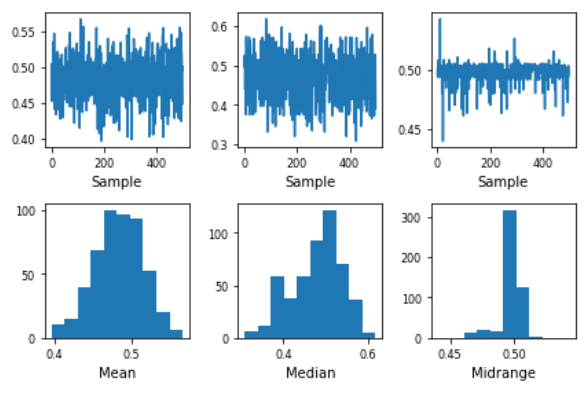# Introduction to Bootstrap plot

• Last Updated : 28 Jun, 2021

Before getting into Bootstrap plot, let us first understand what Bootstrapping (or Bootstrap sampling) is all about.

Bootstrap Sampling: It is a method in which we take a sample data repeatedly with replacement from a data set to estimate a population parameter. It is used to determine various parameters of a population.

Bootstrap plot: It is a graphical method used to measure the uncertainty of any desired statistical characteristic of a population. It is an alternative to the confidence interval. (also a mathematical method used for calculation of a statistic).

Structure

• x-axis: Subsample number.
• y-axis: Computed value of the desired statistic for a given subsample.

Need for a Bootstrap plot:

Commonly, we can calculate the uncertainty of a statistic of a population mathematically, using confidence intervals. However, in many cases, the uncertainty formula that is derived is mathematically intractable. In such cases, we use the Bootstrap plot.

Suppose, we have 5000 people in a park, and we need to find the average weight of the whole population. It is not feasible to measure the weight of each individual and then take an average of that. This is where bootstrap sampling comes into the picture.

What we do is, we take groups of 5 people randomly from the population and find its mean. We do the same process say 8-10 times. This way, we get a good estimate of the average weight of the population more efficiently.

Intuition:

Let us consider an example and understanding how the Bootstrap plot makes it easier to obtain critical information from a large population. Say we have a sample data of 3000 randomly generated uniform numbers. We take out a sub-sample of 30 numbers and find its mean. We do this again for another random sub-sample and so on.

We plot a bootstrap plot of the above-acquired information and just by looking at it, we can easily give a good estimate about the mean of all the 3000 numbers. There is various other useful information one can get out of a bootstrap plot such as:

• which sub-sample had the lowest variance, or
• which sub-sample creates the narrowest confidence interval, etc.

Implementation:

## Python

 `import` `pandas as pd``import` `numpy as np` `s ``=` `pd.Series(np.random.uniform(size``=``500``))``pd.plotting.bootstrap_plot(s)`

OutputLimitation

1. The bootstrap plot gives an estimation of the required information from the population, not the exact values.
2. It is highly dependent on the dataset given. It fails to give good results when a lot of subsets have repeated samples.
3. The bootstrap plot becomes ineffective when we are obtaining information that is highly dependent on the tail values. [As shown in Fig 1]

My Personal Notes arrow_drop_up